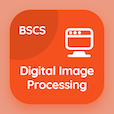Finance Online Courses (BBA)

Financial Management MCQs

Financial Management MCQ PDF - Topics

# Dividend Stock MCQ Quiz Online

Practice Dividend Stock Multiple Choice Questions (MCQ), Dividend Stock quiz answers PDF to learn financial management online course for financial management classes. Stocks Valuation and Stock Market Equilibrium Multiple Choice Questions and Answers (MCQs), Dividend Stock quiz questions for online business university. "Dividend Stock MCQ" PDF Book: common stock valuation, valuing stocks: non constant growth rate, expected rate of return on constant growth stock, dividend stock test prep for online business and management degree.

"The preferred dividend is \$50 and the required rate of return is 2.5% then the value of preferred stock would be" MCQ PDF: dividend stock with choices 0.2, 125, 2000, and 52.5 for online business university. Learn dividend stock quiz questions for merit scholarship test and certificate programs for online school of business administration.

## MCQs on Dividend Stock Quiz

MCQ: The preferred dividend is \$50 and the required rate of return is 2.5% then the value of preferred stock would be

0.2
125
2000
52.5

MCQ: The preferred dividend is divided for required rate of return to calculate

value of number of shares
value of equity
value of preferred stock
value of common stock

MCQ: The value of stock is \$300 and the preferred dividend is \$60 then the required rate of return would be

18000
0.2
20
5

MCQ: The value of stock is \$1200 and the preferred dividend is \$120 then the required rate of return would be

144000
0.1
10
0.2

MCQ: The preferred dividend is \$60 and the required rate of return is 20% then the value of preferred stock will be

40
120
12
300

### More Topics from Financial Management Course